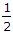# Civil Engineering - Applied Mechanics - Discussion

### Discussion :: Applied Mechanics - Section 5 (Q.No.2)

2.

Pick up the correct statement from the following :

 [A]. If two equal and perfectly elastic smooth spheres impinge directly, they interchange their velocities. [B]. If a sphere impinges directly on an equal sphere which is at rest, then a fraction(1 - e2) the original kinetic energy is lost by the impact. [C]. If a smooth sphere impinges on another sphere, which is at rest, the latter will move along the line of centres. [D]. If two equal spheres which are perfectly elastic impinge at right angles, their direction after impact will still be at right angles. [E]. All the above.

Explanation:

No answer description available for this question.